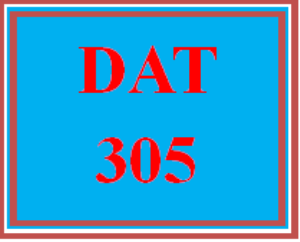# DAT 305 Week 5 Apply - Course Post-Assessment Quiz

PLDZ-12297 In Stock
\$ 15.00 USD
Description

***************************************************************

https://uopcourse.com/category/dat-305/

~~~~~~~~~~~~~~~~~~~~~~~~~~~~~~~~~~~~~~~~~~~~~~~~~

https://uopcourse.com/

***************************************************************

DAT 305 Week 5 Apply – Course Post-Assessment Quiz

Which of the following statements is true?

Removing an element from a linked list is faster than adding an element to a linked list.

Traversing a linked list has the same complexity as removing an element from a list.

Traversing a linked list has the same complexity as adding an element to a list.

Removing and adding an element to a linked list has the same complexity as a traversing operation.

a. 2 and 4

b. 4

c. 1

d. 1 and 3

What is the maximum number of comparisons that can take place in bubble sort? Assume that there are n elements in the array.

a. (1/2)n(n-1)

b. (1/2)(n-1)

c. (1/4)(n-1)

d. (1/4)n(n-1)

What will be the output of the following program?

a. value1

value2

b. A Runtime Exception will be thrown.

c. null

null

d. value2

value2

What will be the output of the following program?

a. null

null

b. value1

value2

c. A Runtime Exception will be thrown.

d. value2

value2

What will be the output of the following program?

a. A NullPointer Exception will be thrown.

b. value1

value2

c. value1

d. value1

null

Which of the following is an advantage of chained hash table (external hashing) over the open addressing scheme?

a. Deletion is easier.

b. Space utilization is less.

c. The worst-case complexity of search operations is less.

d. None of the above.

The given definition describes which of the following terms?

Search or exhaustive search, also known as generate and test, is a very general problem-solving technique and algorithmic paradigm that consists of systematically enumerating all possible candidates for the solution and checking whether each candidate satisfies the problem’s statement.

a. Brute-Force

b. Dynamic programming

c. Greedy algorithm

d. Divide and conquer

Consider the following string: KOTMAALE, and pattern to be matched: AALE. By how many positions will the first shift be performed, using the bad character and good prefix rules?

a. 2

b. 4

c. 1

d. 3

What is a graph without self loops and parallel edges called?

a. A directed cycle graph

b. A cycle graph

c. A complete graph

d. A simple graph

Which of the following are the worst-case running times of insertion sort, merge sort, and quick sort respectively?

a. O(nlog(n)), O(nlog(n)), and O(n^2)

b. O(n^2), O(n log(n)), and O(n log(n))

c. O(n^2), O(n log n), and O(n^2)

d. O(n^2), O(n^2), and O(n log(n))

What is the knapsack value for the given input?

value = [ 20, 5, 10, 40, 15, 25 ]

weight = [1, 2, 3, 8, 7, 4 ]

W = 10

a. 60

b. 35

c. 115

d. 40

Which of the following algorithms is a greedy algorithm?

Merge sort

Quick sort

Insertion sort

Bubble sort

a. Merge sort and quick sort

b. Insertion sort and bubble sort

c. Quick sort and insertion sort

d. None

What data structure is a prime number used with?

a. Hash table

b. Stack

c. Array

d. Queue

For the starting permutation [3, 57, 64, 54, 1, 35, 98], what are the steps to bubble sort into a sorted arrangement of [1, 3, 35, 54, 57, 64, 98]?

a. [1, 3, 35, 54, 57, 64, 98] -> [3, 54, 1, 35, 57, 64, 98] -> [3, 57, 64, 54, 1, 35, 98] -> [3, 1, 35, 54, 57, 64, 98] -> [1, 3, 35, 54, 57, 64, 98] -> [3, 57, 54, 1, 35, 64, 98] -> [1, 3, 35, 54, 57, 64, 98]

b. [3, 1, 35, 54, 57, 64, 98] -> [1, 3, 35, 54, 57, 64, 98] -> [1, 3, 35, 54, 57, 64, 98] -> [3, 57, 64, 54, 1, 35, 98] -> [3, 57, 54, 1, 35, 64, 98] -> [3, 54, 1, 35, 57, 64, 98]

c. [3, 57, 64, 54, 1, 35, 98] -> [3, 57, 54, 1, 35, 64, 98] -> [3, 54, 1, 35, 57, 64, 98] -> [3, 1, 35, 54, 57, 64, 98] -> [1, 3, 35, 54, 57, 64, 98] -> [1, 3, 35, 54, 57, 64, 98]

d. [1, 3, 35, 54, 57, 64, 98] -> [3, 57, 64, 54, 1, 35, 98] -> [3, 1, 35, 54, 57, 64, 98] -> [1, 3, 35, 54, 57, 64, 98] -> [3, 57, 54, 1, 35, 64, 98] -> [3, 54, 1, 35, 57, 64, 98]

How is prime factorization a hard problem?

a. Multiplying prime numbers is difficult

b. Finding the prime factors multiplied for a number is hard

c. Prime factorization uses division

d. Prime factorization is NP-complete

When do we use an adjacency list?

a. For a hash table

b. For a graph that is undirected

c. When sorting a list

d. When searching through a list for an element

Recent Reviews Write a Review
0 0 0 0 reviews